# 1607 Shillelagh

### Today’s Puzzle:

A Shillelagh is an Irish wooden walking stick. This Shillelagh is keeping with our Saint Patrick’s Day theme, but it is a Find the Factors 1 to 14 puzzle.  Brutal! It will be a whole lot less tricky for you to solve because I made it a level 3 puzzle: The logic needed to solve the puzzle is built in. Just start with the clue at the top of the puzzle and work your way down cell by cell until you have found all the factors. So crack on!Print the puzzles or type the solution in this excel file: 14 Factors 1604-1612.

### Factors of 1607:

• 1607 is a prime number.
• Prime factorization: 1607 is prime.
• 1607 has no exponents greater than 1 in its prime factorization, so √1607 cannot be simplified.
• The exponent in the prime factorization is 1. Adding one to that exponent we get (1 + 1) = 2. Therefore 1607 has exactly 2 factors.
• The factors of 1607 are outlined with their factor pair partners in the graphic below.How do we know that 1607 is a prime number? If 1607 were not a prime number, then it would be divisible by at least one prime number less than or equal to √1607. Since 1607 cannot be divided evenly by 2, 3, 5, 7, 11, 13, 17, 19, 23, 29, 31, or 37, we know that 1607 is a prime number.

### More about the Number 1607:

1607 is the sum of two consecutive numbers:
803 + 804 = 1607.

1607 is also the difference of two consecutive numbers:
804² – 803² = 1607.

Did you notice what happened there? Try this next one:

1607² = 2582449.

1607²/2 = 1291224.5.

(1607-1291224-1291225) is a primitive Pythagorean triple.

Cool, isn’t it?

# 1597 and Level 3

### Today’s Puzzle:

You can solve this level 3 puzzle! Each number from 1 to 10 must appear in both the first column and the top row.

What is the greatest common factor of 24 and 56? Write that number above the column in which those clues appear. Write the corresponding factors in the first column. Next, starting with 72, write the factors of each clue going down the puzzle row by row until you have found all the factors.### Factors of 1597:

• 1597 is a prime number.
• Prime factorization: 1597 is prime.
• 1597 has no exponents greater than 1 in its prime factorization, so √1597 cannot be simplified.
• The exponent in the prime factorization is 1. Adding one to that exponent we get (1 + 1) = 2. Therefore 1597 has exactly 2 factors.
• The factors of 1597 are outlined with their factor pair partners in the graphic below.How do we know that 1597 is a prime number? If 1597 were not a prime number, then it would be divisible by at least one prime number less than or equal to √1597. Since 1597 cannot be divided evenly by 2, 3, 5, 7, 11, 13, 17, 19, 23, 29, 31, or 37, we know that 1597 is a prime number.

### More about the Number 1597:

1597 is the 17th Fibonacci number. It is also the only 4-digit Fibonacci prime.

1597 is the sum of two squares:
34² + 21² = 1597.

1597 is the hypotenuse of a Pythagorean triple:
715-1428-1597, calculated from 34² – 21², 2(34)(21), 34² + 21².

Here’s another way we know that 1597 is a prime number: Since its last two digits divided by 4 leave a remainder of 1, and 34² + 21² = 1597 with 34 and 21 having no common prime factors, 1597 will be prime unless it is divisible by a prime number Pythagorean triple hypotenuse less than or equal to √1597. Since 1597 is not divisible by 5, 13, 17, 29, or 37, we know that 1597 is a prime number.

# 1588 Cupid’s Arrow

### Today’s Puzzle:

Keeping with our Valentine’s theme, today’s level 3 puzzle looks like Cupid’s Arrow. Start with the clues at the top of the arrow, write in their factors, and work your way down the puzzle, cell by cell, writing in factors as you go. Before long, you will be smitten with this puzzle!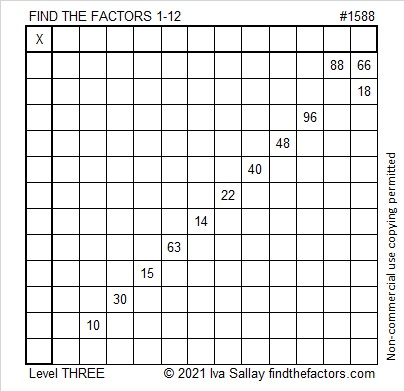### Factors of 1588:

• 1588 is a composite number.
• Prime factorization: 1588 = 2 × 2 × 397, which can be written 1588 = 2² × 397.
• 1588 has at least one exponent greater than 1 in its prime factorization so √1588 can be simplified. Taking the factor pair from the factor pair table below with the largest square number factor, we get √1588 = (√4)(√397) = 2√397.
• The exponents in the prime factorization are 2 and 1. Adding one to each exponent and multiplying we get (2 + 1)(1 + 1) = 3 × 2 = 6. Therefore 1588 has exactly 6 factors.
• The factors of 1588 are outlined with their factor pair partners in the graphic below.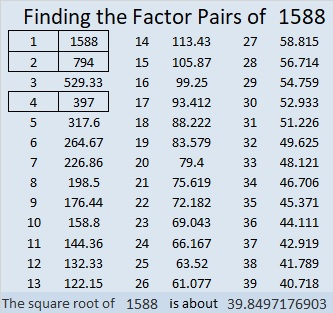### More about the number 1588:

1588 is the difference of two squares:
398² – 396² = 1588.

1588 is also the sum of two squares:
38² + 12² = 1588.

1588 is the hypotenuse of a Pythagorean triple:
912-1300-1588, calculated from 2(38)(12), 38² – 12², 38² + 12².
It is also 4 times (228-325-397).

# 1578 The Logic Needed to Solve This Puzzle is Straight Forward

### Today’s Puzzle:

What is the only common factor of 36 and 9 that will use only numbers from 1 to 10 in the first column? Answer that question, put the factors in their appropriate cells, and then go straight down the puzzle row by row, filling in factors as you go. In no time at all, you will have solved this level 3 puzzle!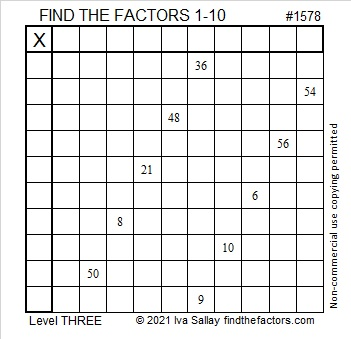### Factors of 1578:

1 + 5 + 7 + 8 = 21, a number divisible by 3, so even number 1578 is divisible by 6.

• 1578 is a composite number.
• Prime factorization: 1578 = 2 × 3 × 263.
• 1578 has no exponents greater than 1 in its prime factorization, so √1578 cannot be simplified.
• The exponents in the prime factorization are 1, 1, and 1. Adding one to each exponent and multiplying we get (1 + 1)(1 + 1)(1 + 1) = 2 × 2 × 2 = 8. Therefore 1578 has exactly 8 factors.
• The factors of 1578 are outlined with their factor pair partners in the graphic below.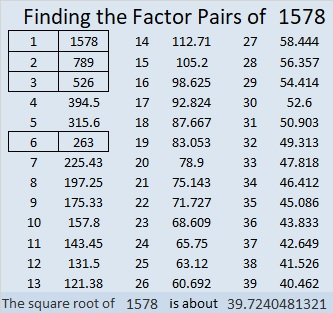### More about the Number 1578:

1578 is a leg in two Pythagorean triples:
1578-622520-622522, calculated from 2(789)(1), 789² – 1², 789² – 1², and
1578-69160-69178, calculated from 2(263)(3), 263² – 3², 263² – 3².

# 1561 Virgács for Boots and Stockings

### Today’s Puzzle:

Children living in Hungary put their nicely polished boots or stockings by a window for Mikulás (Saint Nicholas) to fill tonight. When they awake in the morning, they will find candies, and maybe nuts or fruit to reward them for the good they’ve done this past year. Because even the best children have been at least a little bit naughty sometime during the year, they will also find virgács, gold-painted twigs typically bound together with red ribbon. Now, if a child lives in a place where virgács is not available at the local market, Mikulás could copy today’s virgács puzzle and put it in any boot or stocking left out for him tonight.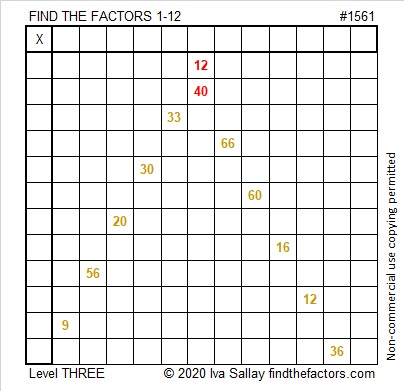Since this is a level 3 puzzle, the clues are listed in a logical order from the top of the puzzle to the bottom. After the factors of 12 and 40 are put in their respective cells, the rest of the factors can be found by working down the puzzle cell by cell until all the factors are written in.

### Factors of 1561:

• 1561 is a composite number.
• Prime factorization: 1561 = 7 × 223.
• 1561 has no exponents greater than 1 in its prime factorization, so √1561 cannot be simplified.
• The exponents in the prime factorization are 1 and 1. Adding one to each exponent and multiplying we get (1 + 1)(1 + 1) = 2 × 2 = 4. Therefore 1561 has exactly 4 factors.
• The factors of 1561 are outlined with their factor pair partners in the graphic below.### More about the Number 1561:

1561 is the sum of two consecutive numbers:
780 + 781 = 1561.

1561 is also the difference of two consecutive square numbers:
781² – 780² = 1561.

Did you notice a pattern in those two statements?

1561 is the sum of seven consecutive numbers:
220 + 221 + 222 + 223 + 224 + 225 + 226 = 1561.

1561 is the sum of the fourteen consecutive numbers from 105 to 118.

1561 is the difference of these two other square numbers:
115² – 108² = 1561.

Did you notice any other patterns? Does your pattern hold true for other multiples of 7?

# 1550 Lucky for You: Solving This Puzzle Is as Easy as Climbing Down a Ladder

### Today’s Puzzle:

It’s Friday the 13th, so don’t walk under any ladders! Still, there isn’t any reason to avoid them entirely. Because this ladder puzzle is a level 3, the clues are given in a logical order to help you find the solution. Start at the top of the ladder, find the common factor of 10 and 18, then work your way down the ladder rung by rung, writing all the numbers from 1 to 10 in both the first column and the top row until you reach the bottom of the ladder.  Good Luck!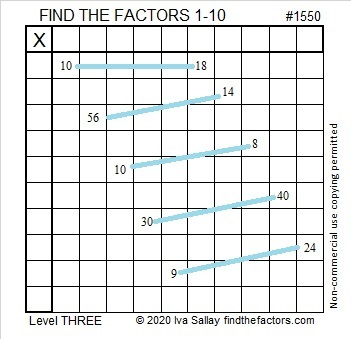Here’s the same puzzle with no colors to distract you.### Factors of 1550:

• 1550 is a composite number.
• Prime factorization: 1550 = 2 × 5 × 5 × 31, which can be written 1550 = 2 × 5² × 31.
• 1550 has at least one exponent greater than 1 in its prime factorization so √1550 can be simplified. Taking the factor pair from the factor pair table below with the largest square number factor, we get √1550 = (√25)(√62) = 5√62.
• The exponents in the prime factorization are 1, 2, and 1. Adding one to each exponent and multiplying we get (1 + 1)(2 + 1)(1 + 1) = 2 × 3 × 2 = 12. Therefore 1550 has exactly 12 factors.
• The factors of 1550 are outlined with their factor pair partners in the graphic below.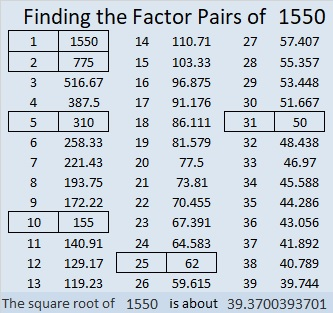### 1550 Factor Tree:

Here’s one of a few possible different factor trees for 1550:### More about the Number 1550:

1550 is the hypotenuse of TWO Pythagorean triples:
434-1488-1550, which is (7-24-25) times 62, and
930-1240-1550, which is (3-4-5) times 310.

# 1538 Second Letter of My Message

### Today’s Puzzle:

This puzzle is the second letter in a message I want to give you. I will give more letters in a few days. How many letters will you need to figure out the message?

I gave you the clues of this level 3 multiplication table puzzle in an order that makes finding the solution easier. It can be solved by finding the common factor of the two clues in the first row of the puzzle and then working your way down the puzzle row by row until you have found all the factors from 1 to 12 for both the first column and the top row.### Factors of 1538:

• 1538 is a composite number.
• Prime factorization: 1538 = 2 × 769.
• 1538 has no exponents greater than 1 in its prime factorization, so √1538 cannot be simplified.
• The exponents in the prime factorization are 1 and 1. Adding one to each exponent and multiplying we get (1 + 1)(1 + 1) = 2 × 2 = 4. Therefore 1538 has exactly 4 factors.
• The factors of 1538 are outlined with their factor pair partners in the graphic below.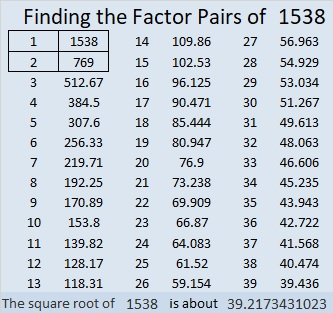### More about the Number 1538:

1538 is the sum of two squares:
37² + 13² = 1538.

1538 is the hypotenuse of a Pythagorean triple:
962-1200-1538 calculated from 2(37)(13), 37² – 13², 37² + 13².

# 1528 Candy Corn

### Today’s Puzzle:

To solve this Level 3 Candy Corn Halloween puzzle, first, find the factors that will work with the clues in the top and bottom rows. Then work you way down row by row filling in factors as you go.

When you get to the 8 in this puzzle, will the factors be 8 × 1 or 4 × 2? Two of those factors will be eliminated because they already appear in the first column. The other two remain possibilities, but one of those factors cannot appear in any other place in that first column, so that is the one you will want to choose. Have a sweet time solving this puzzle!Here is a plain version of the same puzzle: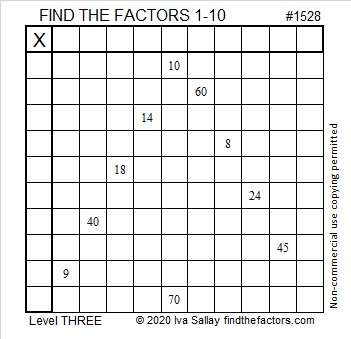### Factors of 1528:

1528 is divisible by two because it is even.

1528 is divisible by four because its last two digits (in the same order) make a number, 28, which is divisible by 4.

Can 1528 be evenly divided by 8? Yes. Here’s a quick way to know: 28 is divisible by 4, but not by 8, AND 5 is odd, so 1528 is divisible by 8, as is every other number ending in 528.

• 1528 is a composite number.
• Prime factorization: 1528 = 2 × 2 × 2 × 191, which can be written 1528 = 2³ × 191.
• 1528 has at least one exponent greater than 1 in its prime factorization so √1528 can be simplified. Taking the factor pair from the factor pair table below with the largest square number factor, we get √1528 = (√4)(√382) = 2√382.
• The exponents in the prime factorization are 3 and 1. Adding one to each exponent and multiplying we get (3 + 1)(1 + 1) = 4 × 2 = 8. Therefore 1528 has exactly 8 factors.
• The factors of 1528 are outlined with their factor pair partners in the graphic below.### Another Fact about the Number 1528:

Since 1528 is divisible by 8 but not by 16, it can be written as the sum of 16 consecutive numbers:
88+89+90+91+92+93+94+95+96+97+98+99+100+101+102+103=1528.

Note that 95 + 96 = 191, and 8 × 191 = 1528.
Likewise, 94 + 97 = 1528,
93 + 98 = 1528, and so forth until we get to…
88 + 103 = 1528.

# 1515 and Level 3

### Today’s Puzzle:

Start with the greatest common factor of 30 and 48, write the factors in the appropriate boxes, then work your way down this level 3 puzzle row by row using logic until you have found all the factors. You can do this!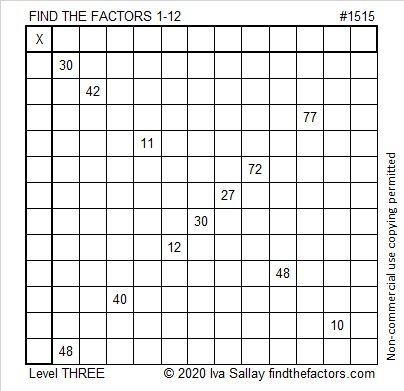### Factors of 1515:

• 1515 is a composite number.
• Prime factorization: 1515 = 3 × 5 × 101.
• 1515 has no exponents greater than 1 in its prime factorization, so √1515 cannot be simplified.
• The exponents in the prime factorization are 1, 1, and 1. Adding one to each exponent and multiplying we get (1 + 1)(1 + 1)(1 + 1) = 2 × 2 × 2 = 8. Therefore 1515 has exactly 8 factors.
• The factors of 1515 are outlined with their factor pair partners in the graphic below.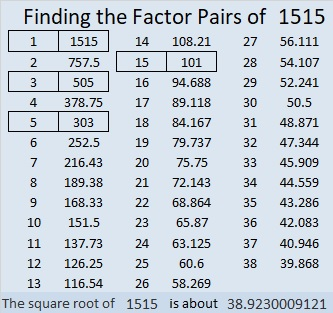### Another Fact about the Number 1515:

1515 is the hypotenuse of FOUR Pythagorean triples:
300-1485-1515, which is 15 times (20-99-101),
651-1368-1515, which is 3 times (217-456-505),
909-1212-1515, which is (3-4-5) times 303,
1008-1131-1515, which is 3 times (336-377-505).

# 1504 and Level 3

### Today’s Puzzle:

Since this is a level 3 puzzle the clues are given in a logical order from top to bottom. Write the factors 1 to 10 in the first column and again in the top row.

Usually, you only have to consider the previous clues when finding the factors in a level 3 puzzle, but when you consider if 4 = 2 × 2 or 1 × 4, you will also have to look at a clue below it. You can do this!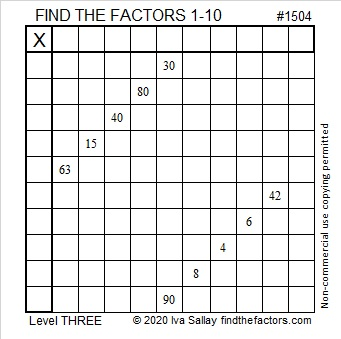### Factors of 1504:

• 1504 is a composite number.
• Prime factorization: 1504 = 2 × 2 × 2 × 2 × 2 × 47, which can be written 1504 = 2⁵ × 47.
• 1504 has at least one exponent greater than 1 in its prime factorization so √1504 can be simplified. Taking the factor pair from the factor pair table below with the largest square number factor, we get √1504 = (√16)(√94) = 4√94.
• The exponents in the prime factorization are 5 and 1. Adding one to each exponent and multiplying we get (5 + 1)(1 + 1) = 6 × 2 = 12. Therefore 1504 has exactly 12 factors.
• The factors of 1504 are outlined with their factor pair partners in the graphic below.### More about the Number 1504:

1504 is the difference of two squares in four different ways:
377² – 375² = 1504
190² – 186² = 1504
98² – 90² = 1504
55² – 39² = 1504